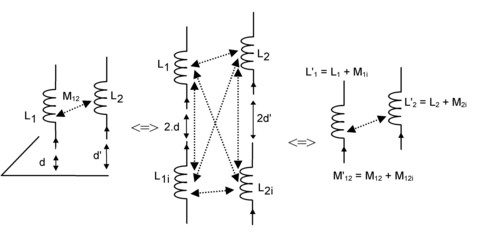# In the presence of a perfect ground plane

## Introduction

The general architecture of structures in power electronics is such that the connection tracks are very often positioned above a conducting plane called a ground plane.

The eddy currents induced in this proximate conducting plane have an influence on the value of partial inductances of these tracks (self-inductance and mutual inductances).

This effect is easily explained by the method of images, as it is presented in the following blocks.

## Method of images: principle

The method of images applies in the presence of a perfect ground plane.

A perfect ground plane is an infinitely extended super-conducting plane (null resistivity)*.

A conductor located at a distance d from a perfect ground plane generates the same structure of the quasi-static magnetic field as two identical parallel conductors placed with respect to one another at the distance 2d in the absence of the ground plane.

If the conductor is parallel to the ground plane, the real conductor and the image conductor contain equal currents of opposite directions (see figure below).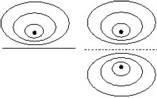* In practice, it is enough that the ground plane exceeds the surface of the circuit.

## Method of images: implemented

In the presence of a ground plane, partial inductances are replaced by equivalent partial inductances in order to take into account the image conductors.

The technique consists in redefining the terminal voltage of each conductor. Examples are presented in the following blocks.

## Partial inductance of a conductor // to a ground plane

The equivalent partial self-inductance of a conductor parallel to a ground plane has the expression: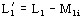where:

• L1 is the self-inductance of the conductor
• M1i is the mutual inductance between the real conductor and its image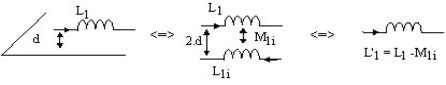## Partial inductance between two // conductors, both // to a ground plane

The equivalent partial mutual inductance between two parallel conductors, both parallel to a ground plane has the expression: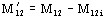where:

• M12 is the mutual inductance between the two conductors
• M12i is the mutual inductance between one of the two conductors and the image of the other one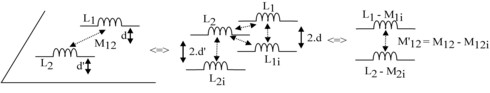## Partial inductance of a conductor ⊥ to a ground plane

The equivalent partial self-inductance of a conductor perpendicular to a ground plane has the expression: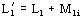where:

• L1 is the self-inductance of a conductor
• M1i is the mutual inductance between the real conductor and its image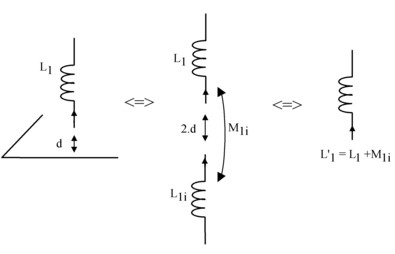## Partial inductance between two // conductors, both ⊥ to a ground plane

The mutual equivalent partial inductance between two parallel conductors, both perpendicular to a ground plane has the expression: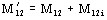where:

• M12 is the mutual inductance between the two conductors
• M12i is the mutual inductance between one of the two conductors and the image of the other one